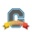New Member

## quizzes

When I add the answer choices for a multiple choice quiz, it asks me for the correct answer which is fine, but when taking the quiz as a dummy student, each question's correct answer was the 1st answer choice.  I checked the box for randomizing the answers and all but the correct answer was affected. How do you change this?